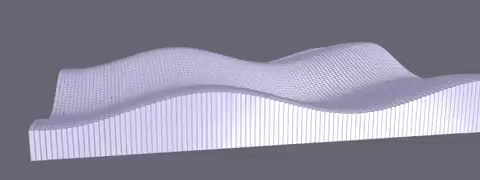API Docs for:

# GeometryBuilder

Module: generation
Parent Module: xeogl

A GeometryBuilder is a builder that you can use to procedurally generate Geometries.## Overview

• Implements the Builder pattern.
• Helps us improve WebGL performance by combining many shapes into the same Geometries, thus reducing the number of draw calls.
• A plain JavaScript class, ie. not a xeogl Component subclass.

## Usage

• Works by accumulating additions to an internal buffer of geometry vertex and index arrays.
• Call setShape() to set its current mesh, and setMatrix() to set its current modelling transform.
• Then, whenever you call addShape(), it appends the shape, transformed by the matrix, to its internal buffer.
• Finally, call build() to dump its buffer into a target Geometry.
• Retains its buffer so that you can call build() again, to dump its buffer into other Geometries as needed.
• Call reset() to empty its buffer.

In the example below we'll use a GeometryBuilder to create something like the screen capture shown above.

``````// Intatiate a GeometryBuilder; note that it's a
// plain JavaScript object, not a xeogl Component subtype
var geometryBuilder = new xeogl.GeometryBuilder();

// Set the current shape we'll be adding to our GeometryBuilder;
// this can be a Geometry, or just an object containing vertex and indices arrays.
geometryBuilder.setShape(new xeogl.BoxGeometry());

// Now add that shape many times, each time setting a different modelling matrix
// on the GeometryBuilder. As we do this, we are accumulating geometry in a buffer
// within the GeometryBuilder.

var matrix = xeogl.math.mat4();
var height = 3;
var height2 = height * 2;
var x;
var y;
var z;
var size = 200;

for (x = -size; x <= size; x += 2) {
for (z = -size; z <= size; z += 2) {

y = ((Math.sin(x * 0.05) * height + Math.sin(z * 0.05) * height)) + height2;

xeogl.math.identityMat4(matrix);            // Fresh matrix
xeogl.math.scaleMat4c(.90, y, .90, matrix); // Post-concatenate scaling
xeogl.math.translateMat4c(x, y, z, matrix); // Post-concatenate translation

geometryBuilder.setMatrix(matrix);          // Set the current modeling transform matrix

geometryBuilder.addShape();                 // Add current shape to the buffer, transformed by the matrix
}
}

var geometry = new xeogl.Geometry();               // Dump the buffer into a Geometry component

geometryBuilder.build(geometry);

var mesh = new xeogl.Mesh({                    // Create an Mesh with our Geometry attached
geometry: geometry,
material: new xeogl.PhongMaterial({
diffuse: [0.6, 0.6, 0.7]
})
});

mesh.scene.camera.eye = [-200, 50, -200];   // Set initial Camera position

new xeogl.CameraControl();                         // Allow camera interaction``````

()

## Methods

### `addShape`

()

Adds a shape to this GeometryBuilder. The shape geometry is the one last added by addShape(), and will be transformed by the matrix that was set by the last call to setMatrix().

A subsequent call to build() will add all the accumulated transformed shapes to a target Geometry.

this

### `build`

()

Returns the accumulated state from previous calls to setMatrix() and setShape().

Retains all that state afterwards, so that you can continue to call this method to add the state to other Geometries.

### `reset`

()

Resets this GeometryBuilder, clearing all the state previously accumulated with setMatrix() and setShape().

this

### `setMatrix`

(
• `matrix`
)

Sets the modeling transform matrix to apply to each shape added with subsequent calls to addShape().

#### Parameters:

• `matrix` Float32Array

a 16-element transform matrix.

this

### `setShape`

(
• `shape`
)

Sets the shape that will be added to this GeometryBuilder on each subsequent call to addShape().

The shape can be either a Geometry or a JavaScript object containing vertex and index arrays.

#### Parameters:

• `shape`